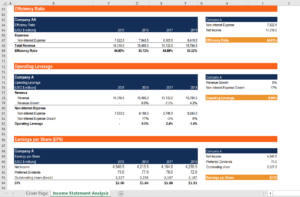# Bank Income Statement Ratio Calculator

Download CFI's free Bank Balance Sheet Ratio Calculator

## Bank Income Statement Ratio Calculator

The Bank Income Statement Ratio Calculator can determine a bank’s profitability, efficiency, and growth through information found on an income statement. Using inputs such as operating expenses, net interest expenses, and net income, the calculator will produce ratios that are important for the analysis of a bank’s income statement.

This template includes the following ratios:

• Efficiency Ratio
• Operating Leverage
• Earnings per Share
• Dividend Yield

Here is a preview of CFI’s Bank Income Statement Ratio Calculator:Click here to download the free template.

### Efficiency Ratio

The efficiency ratio is used to determine the efficiency of a bank by comparing non-interest expense to revenue. The formula for the efficiency ratio is:

Efficiency Ratio = Non-Interest Expense / Revenue

Since the non-interest expense is in the numerator, a higher efficiency ratio suggests a more efficient bank.

### Operating Leverage

Operating leverage is another metric to measure the efficiency of a bank. Unlike the operating leverage used for other companies, calculating this ratio for a bank uses different inputs. For a bank, this ratio compares the growth rate of non-interest expense and revenue.

The formula for the operating leverage is:

Operating Leverage = Growth Rate of Revenue – Growth Rate of Non-Interest Expense

Since the metric takes the difference between the growth rate for revenue and expense, a positive ratio means a bank is more efficient in growing operations.

### Earnings per Share (EPS)

Earnings per share is a metric that is used in all industries. EPS is used to compare the profitability of different companies in the same industry. It is a suitable ratio as allocating earning to the number of shares, the size difference between companies is removed.

The formula for EPS is:

Earnings per Share = (Net Income – Preferred Dividends) / Outstanding Share

A higher EPS demonstrates a company that is more profitable on a shareholder basis.

### Dividend Yield

Dividend yield measures the amount of dividend per share in relation to the share price. This gives investors an easy way of comparing different companies by looking at the return on each dollar spent on a share.

The formula for dividend yield is:

Dividend Yield = Dividend per Share / Share Price

A higher dividend yield demonstrates a higher dividend gained on each dollar spent on purchasing a share.

### Financial Analyst Certification

Become a certified Financial Modeling and Valuation Analyst (FMVA)® by completing CFI’s online financial modeling classes and training program!